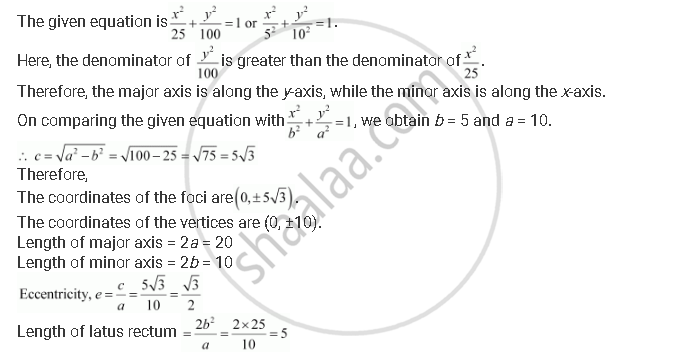CBSE (Arts) Class 11CBSE
Share

# Find the Coordinates of the Foci, the Vertices, the Length of Major Axis, the Minor Axis, the Eccentricity and the Length of the Latus Rectum of the Ellipse X^2/25 + Y^2/100 = 1 - CBSE (Arts) Class 11 - Mathematics

ConceptEllipse Relationship Between Semi-major Axis, Semi-minor Axis and the Distance of the Focus from the Centre of the Ellipse

#### Question

Find the coordinates of the foci, the vertices, the length of major axis, the minor axis, the eccentricity and the length of the latus rectum of the ellipse x^2/25 + y^2/100 = 1

#### SolutionIs there an error in this question or solution?

#### APPEARS IN

Solution Find the Coordinates of the Foci, the Vertices, the Length of Major Axis, the Minor Axis, the Eccentricity and the Length of the Latus Rectum of the Ellipse X^2/25 + Y^2/100 = 1 Concept: Ellipse - Relationship Between Semi-major Axis, Semi-minor Axis and the Distance of the Focus from the Centre of the Ellipse.
S